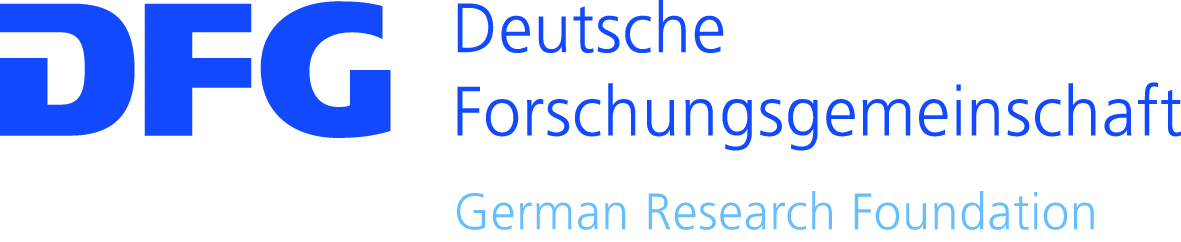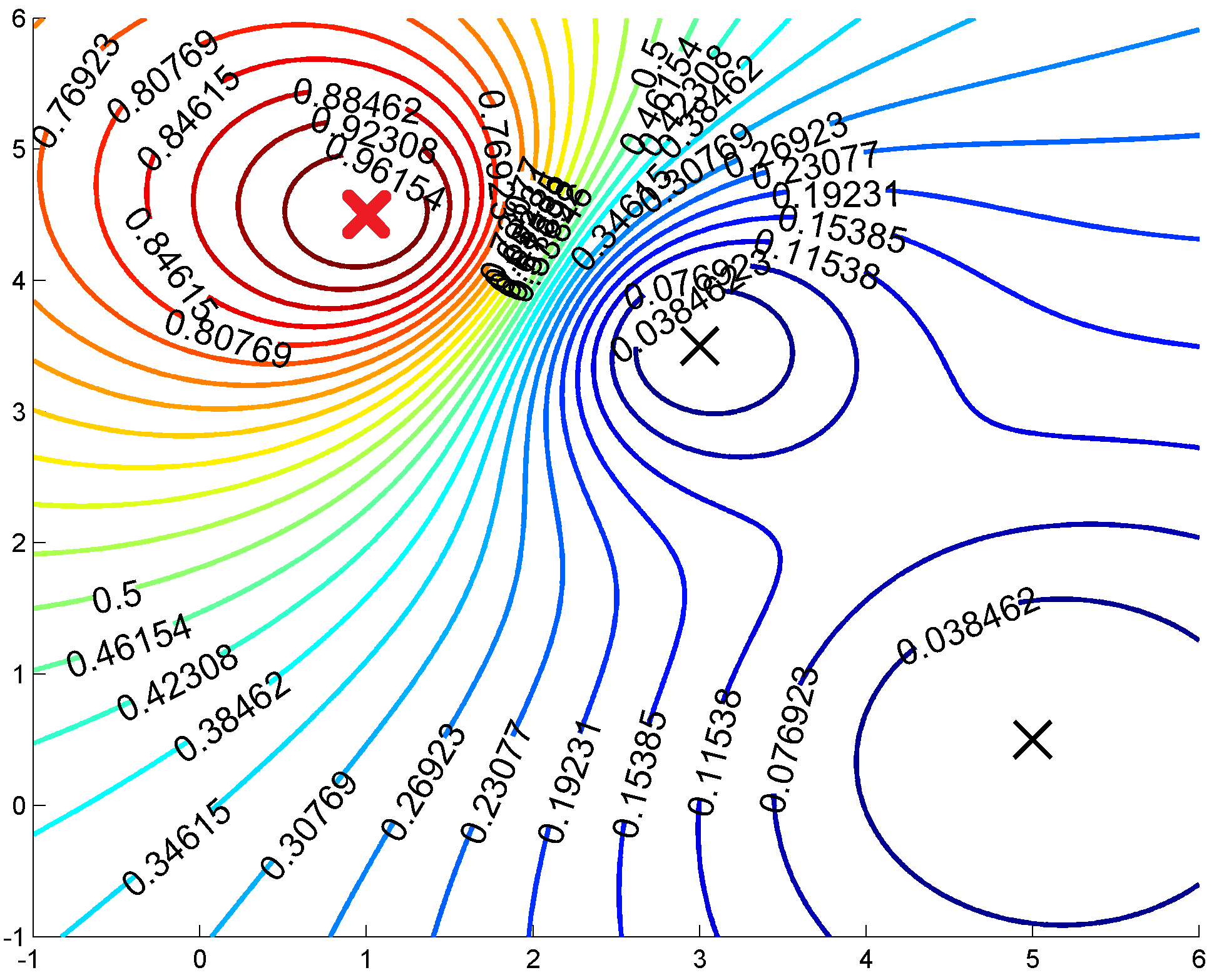# Problem DescriptionA clustering is the partition of some set of objects into groups, the so-called clusters. Computing a good clustering is a well-known problem from machine learning and data mining, with applications is numerous areas, such as data compression, image and signal processing, and pattern recognition. One of the most important partitioning clustering problems is the K-Means problem. However, in practice it is not always sensible to uniquely assign each data point to a single cluster. In contrast, in a soft clustering, each data point belongs to each cluster with a certain degree of membership. A popular soft clustering problem is the Maximum Likelihood Estimation (MLE) problem for mixture models. The Expectation-Maximization (EM) algorithm, which is a generalization of Lloyd's algorithm for the K-Means problem, is a popular tool for solving such an MLE problem. However, a downside of this algorithm is that it is only a heuristic with two significant weaknesses. There are no approximation guarantees for solutions computed by the EM algorithm and there is no known upper bound on its runtime.

# Project GoalThe central goal of this DFG project is the algorithmic and complexity theoretic analysis of the MLE problem for mixture models. This MLE problem has two fundamental structural differences in comparison to the well analyzed K-Means problem. The assignment of points in the form of a soft clustering and the cost function in the form of a mixture model. Our approach is to tackle the MLE problem from two different angles. To this end, we analyze different variants "between" K-Means and the MLE problem. The core idea is that these intermediate problems only exhibit one of the two mentioned structural differences, instead of both at once. In particular, this encompasses the Fuzzy K-Means problem and the CMLE problem. These two are, independent of their role within the context of this project, of high importance to practitioners. Analogously to Lloyd's algorithm and the EM algorithm, there are heuristics which are employed to solve Fuzzy K-Means and CMLE, in practice. We aim to develop new algorithms which solve these problems with a provable approximation ratio and runtime bound. Additionally, we plan to analyze the structural differences between these problems, respectively the (almost) optimal solutions to these problems. This analysis should provide new insight into algorithmic and complexity theoretic aspects of the MLE problem.

## Publications

Coresets for Fuzzy K-Means with Applications
J. Blömer, S. Brauer, K. Bujna, in: 29th International Symposium on Algorithms and Computation  (ISAAC 2018), Schloss Dagstuhl--Leibniz-Zentrum fuer Informatik, 2018, pp. 46:1--46:12.
Complexity of single-swap heuristics for metric facility location and related problems
S. Brauer, Theoretical Computer Science 754 (2019) 88–106.
Classification and Approximation of Geometric Location Problems
S. Brauer, Classification and Approximation of Geometric Location Problems, Paderborn, 2019.
A Complexity Theoretical Study of Fuzzy K-Means
J. Blömer, S. Brauer, K. Bujna, ACM Transactions on Algorithms 16 (2020) 1–25.
How well do SEM algorithms imitate EM algorithms? A non-asymptotic analysis for mixture models
J. Blömer, S. Brauer, K. Bujna, D. Kuntze, Advances in Data Analysis and Classification 14 (2020) 147–173.